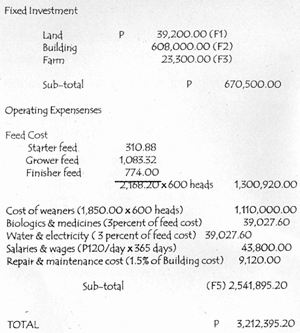# Hog/Swine: Cost Computation and ROIThe most important aim in finisher production is to earn profit. Thus, the farmer must know how to account all the costs involved in the production process to be able to determine how much was gained or lost from his finishing enterprise.

Cost Calculation

Cost is the value of inputs used in the production process. It can be divided into cash and non-cash costs. Cash costs include all out-of-pocket expenses. In computing current prices should be used and all items bought should be properly accounted.

• Example: laborer, feeds, weaners

Non-cash cost include depreciation expense of lasting assets like buildings, farm equipment and tools, if family labor ts employed, the value of his labor is also considered as non-cash cost and this is called opportunity cost of labor or unpaid family labor.

• Example: equipment, pig houses, family labor

How to compute for Cost of Labor

• Cost of Labor = Number of hours devoted to the farm, multiplied by Existing Wage Rate per Pay

Sample Calculation

• 2 hours devoted/family member/day
• 2 family members work in the farm
• P 120/day is the existing wage rate
• 8 working hours/day

Calculate: Total Cost of Labor/year

• (2 hours x 2 persons x 365 days) x P120/8 hours
• 4 man-hours x 365 days x 15
• Cost of Labor/year = P 21,900.00

Depreciation Cost

The Depreciation Cost falls under the non-cash cost. Unlike other costs, it does not result from a cash payment. It arises from expenditures such as buildings, equipment, vehicles, etc. Whenever these assets are used, the value of such items diminishes over time due to depreciation. Once the asset is acquired, it is assumed that it will be used until it wears out. Since the value of an asset has decreased due to wear and tear, it would be unfair to charge the whole acquisition cost of the asset only to the year of operation when it is acquired This is the major justification of charging depreciation cost. After useful years, the value of the asset may finally reduce to zero or a value called scrap or salvage value.

How to compute for the Depreciation Cost

• Annual Depreciation Cost = AC – SV / n

where:

• AC = Acquisition Cost
• SV = Salvage Value
• n = Lifespan

Sample Calculation

Calculate the depreciation cost of a wheelbarrow with an acquisition cost of P800 with a zero salvage value and expected to last for 4 years.

• Annual Depreciation Cost = 800 – 0/4 years
• P200/year

The value of the wheel barrow is decreasing by P 200/yr, this also means that after using it for (our years, the value of the farm tool is zero.

Cost and Return Analysis

The Cost and Return Analysis is the most common method of determining the profitability of a farm business. The Net Return is also referred to as the Net Income. Net Return is the profit from the year’s operation and represents the return to the owner for persona 1 labor, management, and equity used in the farm.

Before making a complete budget for the establishment of a finisher farm, it is important to identify the technical and financial starting points. These starting points will be used in the Cost and Return Analysis of your farm.

Technical Starting Points

• Number of Finishers Per Batch – 200
• Starting weight of weaner (kg) – 20
• Market weight (kg) – 90
• Fattening days (includes cleaning & disinfection) – 120
• Average Daily Gain (g) – 583
• Feed Conversion Ratio – 2.94

Feed consumption/finisher:

• Starter feed (kg) – 26.80
• Grower feed (kg) – 102.20
• Finisher feed (kg) – 77.40
• Total – 206.40

Number of rounds per year – 3
Mortality – 3%
Number of finishers produced per year – 582

 Financial Starting Points Total Cost Required to Establish a 200 Fattener ProductionNet Return = Total Return/year – Total Costs/year

Total Return

Cash Return Sale

• Finishers (582 heads x 90 x P60/kg LW) – P3,142,800.00
• Empty sacks (2,477 pcs x P5) – P12,385
• Total Return – P 3,155,185.00

Total Cost/Year

Cash Cost – P 2,541,895.00 (F5)

Non-cash cost (Depreciation expense)

• Building – 36,480.00
• Equipment – P2,673.75
• Sub-Total – P39,153.75

Total Cost – (2,620,202.40)

Net Return/Year – P634,982.60

Cost Price

The Cost Price is always based on the volume of production considering all costs incurred in the production process. It is needed to be able to determine the break-even point. A value lower than the cost price indicate loss while a value higher means profit.

• Cost Price = Total Cost of Production/year, divided by Number of Finishers marketed/year

Sample Computation

• Total Cost of production year P 2,581,048.95
• Number of finishers marketed/year 582

Cost Price = P2,581,048.95 / 582

• P 4,434.79/ 90kg finisher or 4929 kg LW

Less than P49.29/kg LW indicates loss and greater than this figure means profit.

Return on Investment (ROI)

Return on Investment is a measure of profitability relative to the total capital invested in the business.

Sample Calculation

• Net Return (Total Return – Total Cost) – 674,136.05
• Total Fixed Assets – 670,850.00
• Cash Costs (Total Working Expenses) – 2,541,895.20

ROI

• = 674,136.05 / (670,850.00 + 2,541,895.20) x 100
• = 674,136.05 / 3,212,745.20 x 100
• = 21%

Payback Period = 100 (constant) / 21 = 4.7 years

The 21 percent ROI means that for every peso invested, there is a 21 centavo return. The higher the ROI, the shorter would be the payback period- This means that it would take the farmer 4.7 years to recover the invested money in his pig raising business. The shorter the payback period, the better the financial status of the business.

source: DA-International Training on Pig Husbandry